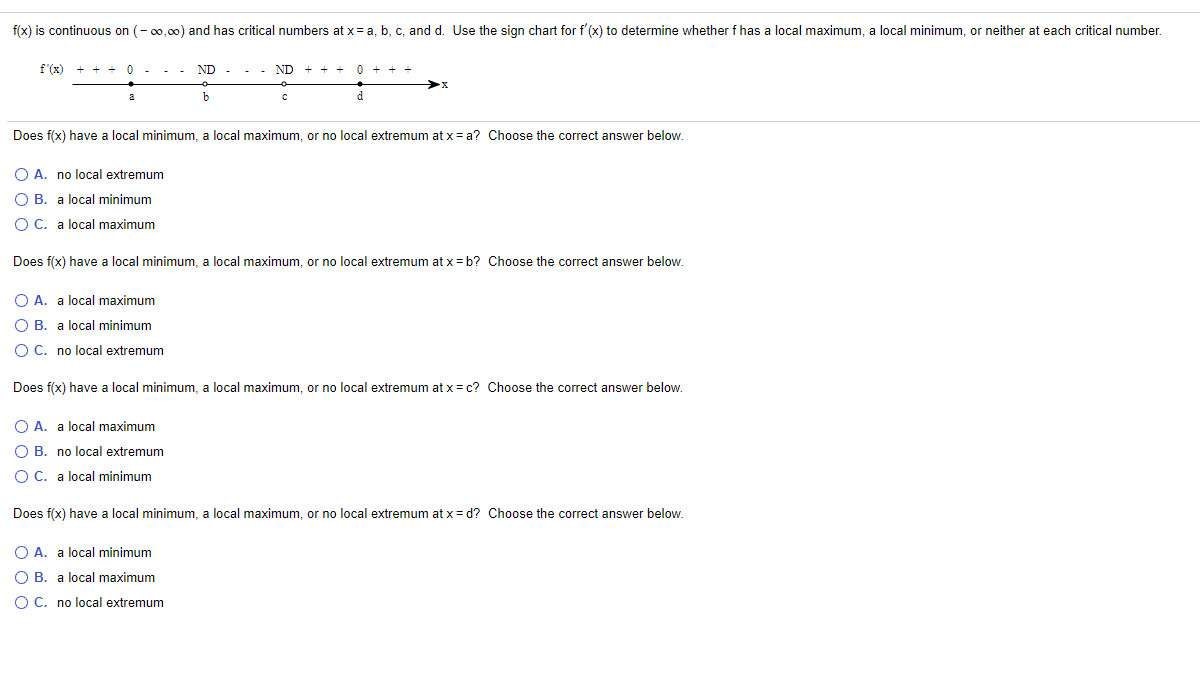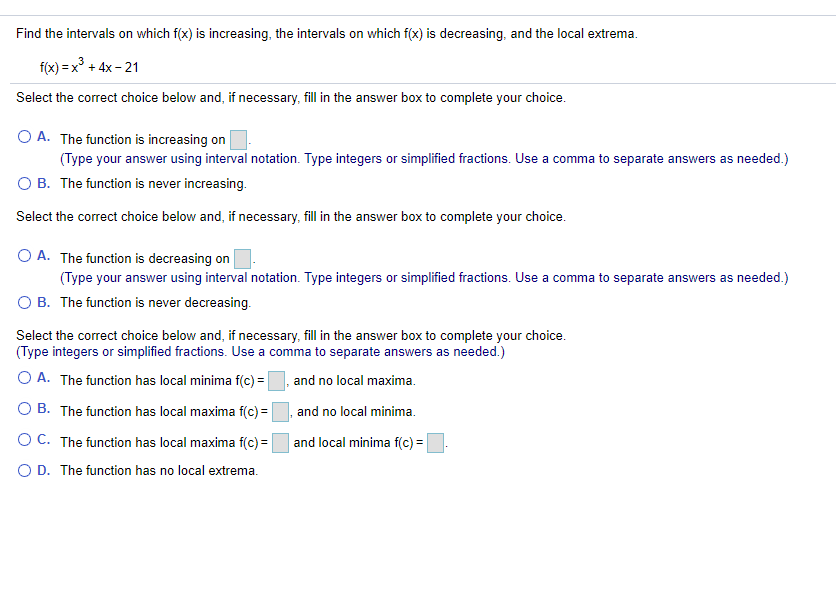### Create an Account

Already have account?

### Forgot Your Password ?

Home / Questions / f(x) is continuous on (-00,00) and has critical numbers at x = a, b, c, and d. Use the sig...

# f(x) is continuous on (-00,00) and has critical numbers at x = a, b, c, and d. Use the sign chart for f'(x) to determine whether f has a local maximum, a local minimum, or neither at each critical num

f(x) is continuous on (-00,00) and has critical numbers at x = a, b, c, and d. Use the sign chart for f'(x) to determine whether f has a local maximum, a local minimum, or neither at each critical number. f'(x) ND. ND a b c d Does f(x) have local minimum, a local maximum, or no local extremum at x=a? Choose the correct answer below. O A. no local extremum OB. a local minimum O C. a local maximum Does f(x) have local minimum, a local maximum, or no local extremum at x = b? Choose the correct answer below. O A. a local maximum OB. a local minimum O C. no local extremum Does f(x) have a local minimum, a local maximum, or no local extremum at x = c? Choose the correct answer below. O A. a local maximum OB. no local extremum O C. a local minimum Does f(x) have a local minimum, a local maximum, or no local extremum at x = d? Choose the correct answer below. O A. a local minimum O B. a local maximum O C. no local extremum
Find the intervals on which f(x) is increasing, the intervals on which f(x) is decreasing, and the local extrema. f(x)=x2 + 4x - 21 Select the correct choice below and, if necessary, fill in the answer box to complete your choice. O A. The function is increasing on (Type your answer using interval notation. Type integers or simplified fractions. Use a comma to separate answers as needed.) O B. The function is never increasing. Select the correct choice below and, if necessary, fill in the answer box to complete your choice. O A. The function is decreasing on (Type your answer using interval notation. Type integers or simplified fractions. Use a comma to separate answers as needed.) OB. The function is never decreasing. Select the correct choice below and, if necessary, fill in the answer box to complete your choice. (Type integers or simplified fractions. Use a comma to separate answers as needed.) O A. The function has local minima f(c) = and no local maxima. OB. The function has local maxima f(c) = and no local minima. OC. The function has local maxima f(c) = and local minima f(c) = OD. The function has no local extrema.Apr 14 2021 View more View Less

#### Answer (Solved)Subscribe To Get Solution